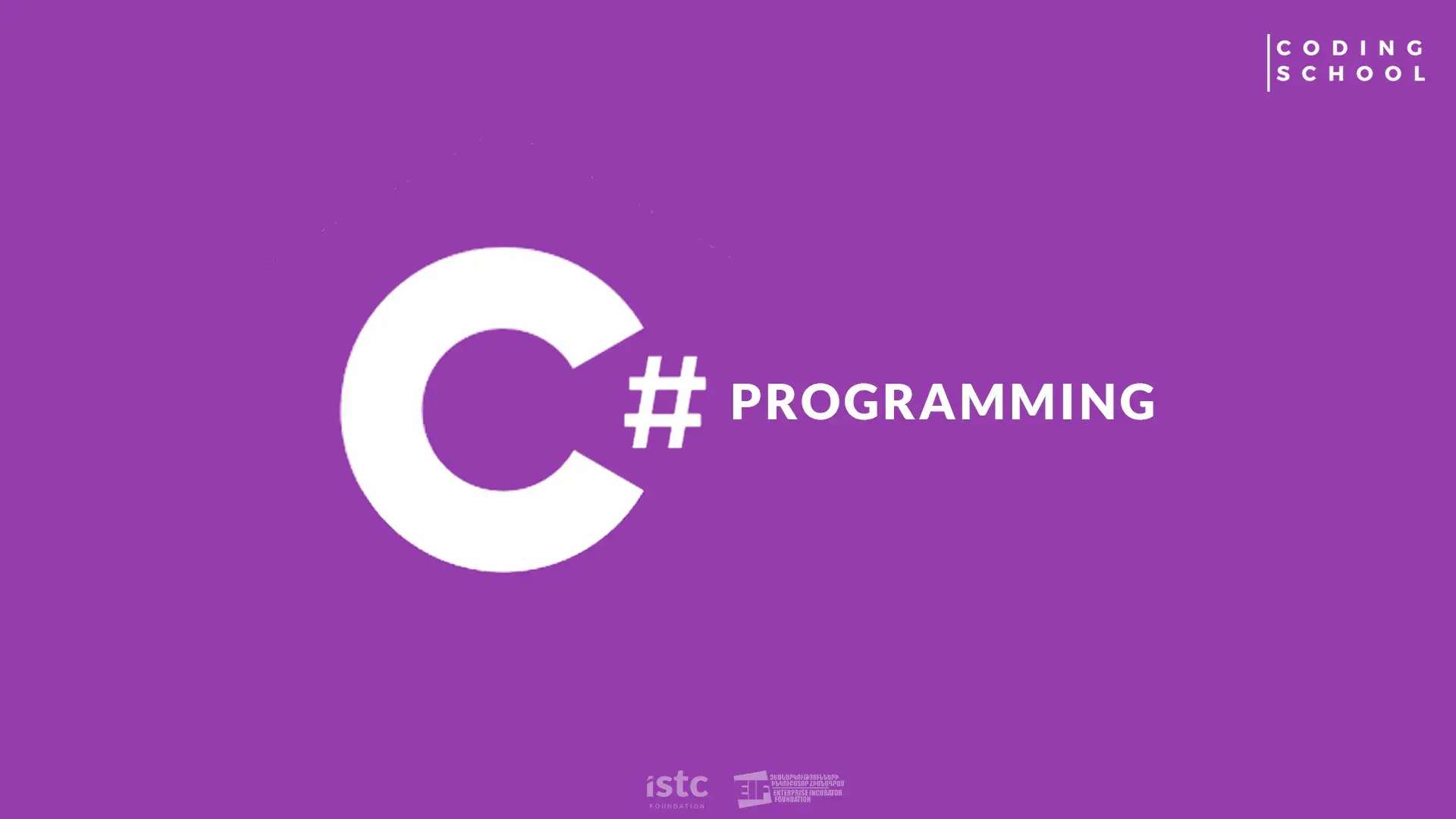# Break It Down

Thursday, 14 March 2019I was recently refactoring a project that relied on a single function to handle a myriad of API calls and database gets and sets. It was, and still is an impressive piece of work and functions perfectly well. As a member of the Freeze Team I have been learning about the `Single Responsibility Principle` and when I came across this function I found it a great opportunity to practice what I've learned.

``````export function startTheProcess(data) {  // The Beginning, the first step is to
dispatch(reduxFlag);              // take the data, send it via Axios.
DataObject = data;
axios.post(send DataObject)
.then(response => {
return DataObject.Info = doStuff(response) // Manipulate the return and
})                                            // create another dataObject
.then(DataObject.Info => {                    // with that info.
DataObject = doStuff(DataObject.Info);
axios.post(send DataObject)            // Then make another Axios Post
.then(response => {                 // with the new data object.
return DataObject = doStuff(response)
})
.then(DataObject => {                    // Then manipulate the return
dispatch(reduxFlag)                    // and create the final
doStuff(DataObject);                   // dataObject and set the in
setDatabaseWith(DataObject)            // the database.
}).catch(err => dispatch(error(err)))    // Complete!

})
.catch(err =>
dispatch(error(err)))
}
``````

Now this example is a little more vague and a lot shorter than the original. But as can be observed, there are several areas that could be optimized using the "single responsibility principle".

My basic plan of attack was to refactor every "then" into it's own function, add a dash of semantic labeling and take it from there. My idea was to create a waterfall of functions, with each one creating the data necessary for, and calling the next.
While this is not adhering strictly to the "single responsibility principle" I feel it is a step in the right direction. I ended up with something like this:

``````// This is the start of the process. It takes the inital 'A' data and sends that to // the first function.

export function runProcess(data) {
try {
dispatch(startFlag);
makeDataObjectA(data);
}
catch(err){
dispatch(error(err))
}
}

async function makeDataObjectA(dataForObjectA){

//  This makes the first DataObjectA, the simple way.
let dataObjectA = dataForObjectA;

//  makes the Axios Post and passes the return to a helper function
let DataObjectA = await axios.post(dataObjectA);
let dataObjectBinfo = getDataObjectInfo(DataObjectA);
//  and then calls the next function in the line with those results.
makeDataObjectB(dataObjectBinfo);
}

// I follow the previous pattern once again

async function makeDataObjectB(dataForObjectB){
let DataObjectB = await axios.post(send dataObjectBinfo)
let dataObjectCinfo = getDataObjectInfo(dataObjectBinfo);
makeDataObjectC(dataObjectCinfo)
}

// The final function that sets the database and triggers the complete flag.

function makeDataObjectC(dataForObjectC) {
let DataObjectC = dataForObjectC;
DataBase.Set(DataObjectC)
dispatch(finshedFlag)
}

// This is the Helper function

function getDataObjectInfo(dataObject){
let ObjectInfo = evaluates from dataObject
return ObjectInfo;
}
``````

A longer read, but easier to get context, easier to debug and a little clearer.